A solid conducting sphere of radius a having a charge $\mathrm{q}$ is surrounded by a concentric conducting spherical shell of inner radius $2\mathrm{a}$ and outer radius $3\mathrm{a}$ as shown in figure.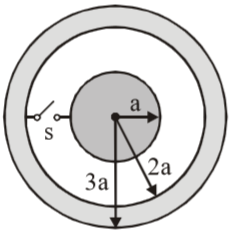Find the amount of heat produced when switch is closed.

1. ${\mathrm{Kq}}^{2}/2\mathrm{a}$

2. ${\mathrm{Kq}}^{2}/3\mathrm{a}$

3. ${\mathrm{Kq}}^{2}/4\mathrm{a}$

4. ${\mathrm{Kq}}^{2}/6\mathrm{a}$

Concept Questions :-

Combination of capactiors
High Yielding Test Series + Question Bank - NEET 2020

Difficulty Level:

Three uncharged capacitors of capacities  and ${\mathrm{C}}_{3}$ are connected to one another as shown in the figure.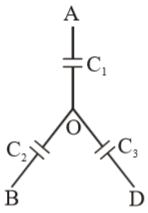Points A, B, and D are at potential  and ${\mathrm{V}}_{3}$ ,then the potential at O will be

1. $\frac{{\mathrm{V}}_{1}{\mathrm{C}}_{1}+{\mathrm{V}}_{2}{\mathrm{C}}_{2}+{\mathrm{V}}_{3}{\mathrm{C}}_{3}}{{\mathrm{C}}_{1}+{\mathrm{C}}_{2}+{\mathrm{C}}_{3}}$

2. $\frac{{\mathrm{V}}_{1}+{\mathrm{V}}_{2}+{\mathrm{V}}_{3}}{{\mathrm{C}}_{1}+{\mathrm{C}}_{2}+{\mathrm{C}}_{3}}$

3. $\frac{{\mathrm{V}}_{1}\left({\mathrm{V}}_{2}+{\mathrm{V}}_{3}\right)}{{\mathrm{C}}_{1}\left({\mathrm{C}}_{2}+{\mathrm{C}}_{3}\right)}$

4. $\frac{{\mathrm{V}}_{1}{\mathrm{V}}_{2}{\mathrm{V}}_{3}}{{\mathrm{C}}_{1}{\mathrm{C}}_{2}{\mathrm{C}}_{3}}$

Concept Questions :-

Combination of capactiors
High Yielding Test Series + Question Bank - NEET 2020

Difficulty Level:

A parallel plate capacitor of area ‘A’ , plate separation ‘d’ is filled with two dielectrics as shown. What is the capacitance of the arrangement ?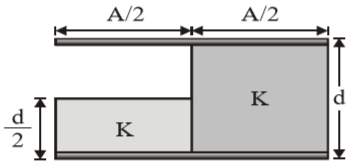1.  $\frac{3{\mathrm{K\epsilon }}_{0}\mathrm{A}}{4\mathrm{d}}$

2. $\frac{4{\mathrm{K\epsilon }}_{0}\mathrm{A}}{3\mathrm{d}}$

3. $\frac{\left(\mathrm{K}+1\right){\mathrm{\epsilon }}_{0}\mathrm{A}}{2\mathrm{d}}$

4.$\frac{\mathrm{K}\left(\mathrm{K}+3\right){\mathrm{\epsilon }}_{0}\mathrm{A}}{2\left(\mathrm{K}+1\right)\mathrm{d}}$

Concept Questions :-

Capacitance
High Yielding Test Series + Question Bank - NEET 2020

Difficulty Level:

Assertion : A particle $\mathrm{A}$ of mass m and charge $\mathrm{Q}$ moves directly towards a fixed particle $\mathrm{B}$, which

has charge $\mathrm{Q}$. The speed of $\mathrm{A}$ is $\mathrm{v}$ when it is far away from $\mathrm{B}$. The minimum separation

between the particles is proportional to ${\mathrm{Q}}^{2}$.

Reason : Total energy remains conserved.

1. If both the assertion and the reason are true and the reason is a correct explanation of the assertion
2. If both the assertion and reason are true but the reason is not a correct explanation of the assertion
3. If the assertion is true but the reason is false
4. If both the assertion and reason are false

Concept Questions :-

Electric potential energy
High Yielding Test Series + Question Bank - NEET 2020

Difficulty Level:

Assertion : Potential difference between two points lying in a uniform electric field may be equal

to zero.

Reason : Points of line normal to electric field is equipotential line.

1. If both the assertion and the reason are true and the reason is a correct explanation of the assertion
2. If both the assertion and reason are true but the reason is not a correct explanation of the assertion
3. If the assertion is true but the reason is false
4. If both the assertion and reason are false

Concept Questions :-

Equipotential surfaces
High Yielding Test Series + Question Bank - NEET 2020

Difficulty Level:

Assertion :  At a distance r from a +ve charged particle potential is V and electric field strength is E.

Then graph between ${\mathrm{V}}^{2}$$\mathrm{E}$ is straight line.

Reason : For a point charge $\frac{{\mathrm{V}}^{2}}{\mathrm{E}}=\frac{\mathrm{q}}{4\mathrm{\pi }{\in }_{0}}$

1. If both the assertion and the reason are true and the reason is a correct explanation of the assertion
2. If both the assertion and reason are true but the reason is not a correct explanation of the assertion
3. If the assertion is true but the reason is false
4. If both the assertion and reason are false

Concept Questions :-

Relation between field and potential
High Yielding Test Series + Question Bank - NEET 2020

Difficulty Level:

Assertion : Electrons move away from a region of lower potential to a region of higher potential.

Reason : Since an electron has a negative charge.

1. If both the assertion and the reason are true and the reason is a correct explanation of the assertion
2. If both the assertion and reason are true but the reason is not a correct explanation of the assertion
3. If the assertion is true but the reason is false
4. If both the assertion and reason are false

Concept Questions :-

Electric potential
High Yielding Test Series + Question Bank - NEET 2020

Difficulty Level:

Assertion : An isolated system consists of two particles of equal masses m=10 gm and charges

${\mathrm{q}}_{1}=+1µ\mathrm{C}$ and  as shown in figure. The initial separation of both charges

is $\mathcal{l}$= 1m. Both the charges are given initial velocities  and  towards

right. Then the maximum separation between the charges is infinite.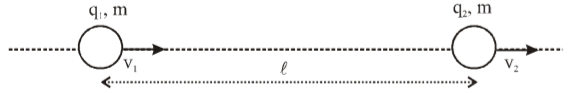Reason : The total energy (Kinetic energy + electrostatic potential energy) of given two particle

system is positive and initial velocity of separation is positive.

1. If both the assertion and the reason are true and the reason is a correct explanation of the assertion
2. If both the assertion and reason are true but the reason is not a correct explanation of the assertion
3. If the assertion is true but the reason is false
4. If both the assertion and reason are false

Concept Questions :-

Electric potential energy
High Yielding Test Series + Question Bank - NEET 2020

Difficulty Level:

Assertion : Charges are given to plates of two plane parallel plate capacitors ${\mathrm{C}}_{1}$ and ${\mathrm{C}}_{2}$ (such

that ${\mathrm{C}}_{2}=2{\mathrm{C}}_{1}$) as shown in figure. Then the key $\mathrm{K}$ is pressed to complete the circuit.

Finally the net charge on upper plate and net charge on lower plate of capacitor ${\mathrm{C}}_{1}$ is positive.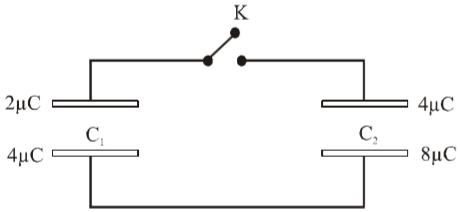Reason : In a parallel plate capacitor both plates always carry equal and opposite charge.

1. If both the assertion and the reason are true and the reason is a correct explanation of the assertion
2. If both the assertion and reason are true but the reason is not a correct explanation of the assertion
3. If the assertion is true but the reason is false
4. If both the assertion and reason are false

Concept Questions :-

Capacitance
High Yielding Test Series + Question Bank - NEET 2020

Difficulty Level:

Assertion :  Each of the plates of a parallel-plate capacitor is given equal positive charge Q. The

charges on the facing surfaces will be same.

Reason : A negative charge (–Q) will be induced on each of the facing surfaces.

1. If both the assertion and the reason are true and the reason is a correct explanation of the assertion
2. If both the assertion and reason are true but the reason is not a correct explanation of the assertion
3. If the assertion is true but the reason is false
4. If both the assertion and reason are false

Concept Questions :-

Capacitance